Trigonometry: Double-Angle and Half-Angle Formulas

Double-Angle Formulas2 mm2 mm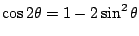Half-Angle Formulas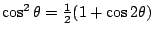, so, so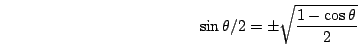Ex 1 Findusing a half-angle formula.

Sol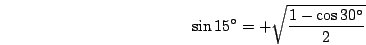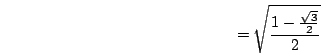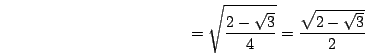Ex 2 Findifand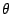is in Quadrant II.

Sol We have that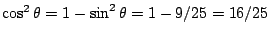, so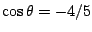sincein Quadrant II. Therefore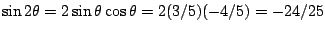.

Pr A Simplify the expression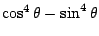.

Pr 1 Findif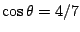.

Pr 2 Findifand.

Pr 3 Use a half-angle formula to find.

Pr 4 Simplify the expressionPr 5 Use half-angle formulas to rewritewithout using powers of trig functions.

Pr 6 Use half-angle formulas to rewritewithout using powers of trig functions.

Pr 7 Findif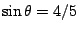and.

Pr 8 Findif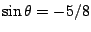and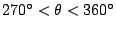.

Go to Solutions.